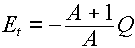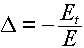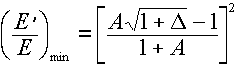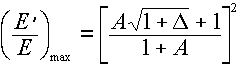# How to calculate/get info about inelastic scattered neutron?

I have been researching in the Forum, but I did not see anything conclusive about this: sorry if that was posted anywhere else here, I am new here.

The energy loss of a neutron after an elastic scattering is a pretty straightforward calculation that can be done by hand. However, I am straggling to get information about how to calculate the same for an inelastic scattering, or an online database where I can find such information.

I would be interested to know which is the resulting energy of a neutron which scatters light nucleai like 19F, 16O or 9Be at MeV level. For instance, 14MeV neutron colliding to 19F: 19F(n,n'), which is the final energy of the collided neutron n'?

Update:

Ok, I think I got it. I found this interesting resource: http://web.utk.edu/~rpevey/NE406/lesson8.htm

It looks like important is to know the first excited state of the nuclei after the scattering with an incident neutron with an energy E. This 1st excited state gives the Q-value, which is used to calculate the energy threshold of the inelastic scatter interaction:Knowing Et and E the ratio Δ between them is calculated as:And then an energy interval for the inelastically scattered neutron can be calculated:The excitation states of the nuclides can be found here: http://www.nndc.bnl.gov/chart/

So if I did not understand something wrong this looks also pretty straightforward